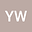Existence of solutions for fractional $m$-point boundary-value problems at resonance with $p$-Laplacian operator
•In this paper, we considered a class of $m$-point boundary-value problem of fractional differential equations at resonance with $p$-Laplacian operator in the following: \begin{eqnarray*} \left\{ \begin{array}{ll} D_{0^+}^\beta \varphi_p (D_{0^+}^\alpha u(t)) = f(t,u(t),D_{0^+}^{\alpha - 2} u(t),D_{0^+}^{\alpha - 1} u(t), D_{0^+}^\alpha u(t)),\quad t \in (0,1), \\ u(0) = u’(0)=D_{0^+}^\alpha u(0) = 0,\quad D_{0^+}^{\alpha - 2} u(1) = \sum_{i = 1}^{m-2} {a_i D_{0^+}^{\alpha - 2} u(\eta_i )} , \end{array} \right. \end{eqnarray*} where $2 < \alpha \le 3$, $\eta_1 <\eta_2 <\cdots< \eta_{m-2}$, $0 < \beta \le 1$, $3 < \alpha + \beta \le 4$, $\sum_{i = 1}^{m-2}a_i\eta_i = 1$, $D_{0^+}^\alpha$ denote the Riemann-Liouville fractional derivative, $\varphi_{p}(s)=|s|^{p-2}s$ is $p$-Laplacian operator. The existence of solutions to above problem is obtained by using the extension of Mawhin’s continuation theorem. It is note that our method dropped a usual condition in the process of investigating above problem. So, in some sense, we got a new result under weaker condition than previous ones\cite{st}.